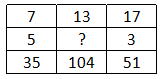Question 17

# In the following question, select the missing number from the given series.Solution

The number at the end in each column is obtained by multiplying the other two.

Eg :- $$7\times5=35$$ and $$17\times3=51$$

Similarly, $$13\times x=104$$

=> $$x=\frac{104}{13}=8$$

=> Ans - (A)